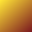#F = 9/5 C + 32

We say that temperature reported in Fahrenheit would be nine fifths that in Celsius plus 32 degrees.

We could divide 9 by 5 to get a ratio.

We could multiply Celsius (C) by a ratio.

We could add 32 to a product to get Fahrenheit (F).

Some languages will compute with fractional precision when constants are written with decimal points. We would write the ratio nine fifths as 9.0/5.0.

Most languages will allow you to be very specific about the order of operations by adding parentheses. Being careful, we could say F = ((9.0/5.0) * C) + 32.0

Multiplication and division are usually done before addition and subtraction. Know when your language don't do operator precedence the usual way.

Some languages will discard any remainder when dividing numbers known to be integers. In this case the ratio 9/5 would divide out to be just 1, not what we want.

If we wanted Fahrenheit to within a degree then we could compute it using just integers so long as we chose a shrewd order of operations.

Some languages offer a special operator for integer division. This might be div or //.

If we are careful to multiply before dividing we can convert C to F using integers like this, F = ((9 * F) // 5) + 32.

Results computed with integers are often truncated to the largest integer less than or equal to the mathematical result. This is not the preferred kind of rounding which would ask for the integer closest to the mathematical result.

We can get the more preferred rounding by adding a bias to the product before doing the truncating divide. If we say, F = ((18 * F) + 5) // 10) + 32, then the +5 represents a bias of 0.5 after the divide, just what we want.